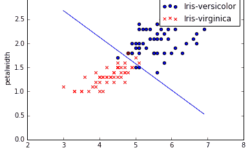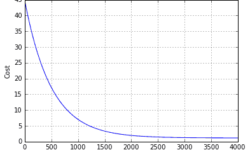## Implementing logistic regression learner with pythonLogistic regression is the next step from linear regression. The most real-life data have a non-linear relationship; thus, applying linear models might be ineffective. Logistic regression is capable of handling non-linear effects in prediction tasks. You can think of many different scenarios where logistic regression could be applied. There can be financial, demographic, health, weather, and other data where the model could be implemented and used to predict subsequent events on future data. For instance, you can classify emails into spam and non-spam, transactions being a fraud or not, and tumors being malignant or benign. In order to understand logistic regression, let’s cover some basics, do a simple classification on data set with two features, and then test it on real-life data with multiple features.

## Linear regression with multiple featuresSingle feature linear regression is simple. All you need is to find a function that fits training data best. It is also easy to plot data and learning curves. But in reality, regression analysis is based on multiple features. So in most cases, we cannot imagine the multidimensional space where data could be plotted. We need to rely on the methods we use. You must feel comfortable with linear algebra, where matrices and vectors are used. If previously we had one feature (temperature), now we need to introduce more of them. So we need to expand hypothesis to accept more features. From now and later on, instead of output y, we are going to use h(x) notation: As you can see, with more variables (features), we also end up with more parameters θ that has to be learned. Before we move let’s find relevant data that we could use for building machine learning algorithm. The data set Again we are going to use data set college cengage. This time we select health data set with several variables. The data (X1, X2, X3, X4, X5) are by city. X1 = death rate per 1000 residents X2 = doctor availability per 100,000…